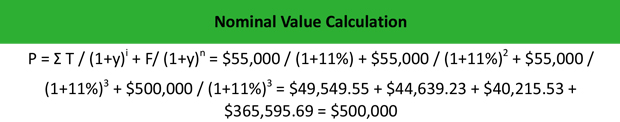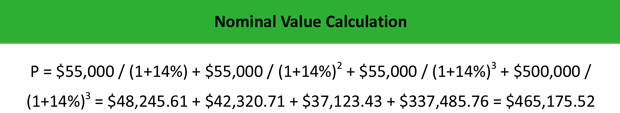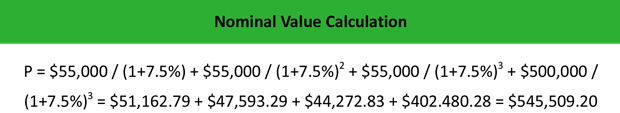# What is Nominal Value?

Definition: Nominal value, also known as face value or par value, is the stated price written on front of a security, usually a bond or stock. For a bond or preferred stock, this is the redemption price or the amount of money that the bondholder will receive at maturity.

## What Does Nominal Value Mean?

What is the definition of nominal value? The NV is the specified value of an issued security, a bond or stock that does not change until maturity, as opposed to the security’s market value that changes based on the impact of the inflation. Normally, the corporate bonds have a value at maturity of \$1,000; whereas, municipal bonds, or muni bonds, are issued at \$5,000 each. Government bonds, however, are typically issued at \$10,000 each.

When the yield to maturity equals the nominal interest rate, the face value equals the value of the bond. When the yield to maturity exceeds the nominal interest rate, the face value is greater than the value of the bond. When the yield to maturity is lower than the nominal interest rate, the face value is lower than the price of the bond.

Let’s look at an example.

## Example

Company Z issues a 3-year bond with a nominal value of \$500,000 and a nominal rate of 11 %. Peter, who works as a financial analyst at the company, wants to calculate at what price the company has to sell the bond if the market rate equals the following:

• Bond #1: 11%
• Bond #2: 14%
• Bond #3: 7.5%

Through maturity, the bond returns \$500,000 x 11% = \$55,000 per year.

The price of the bond when the nominal rate i=11% and yield to maturity y=11% is:The price of the bond when the nominal rate i=11% and yield to maturity y=14% is:The price of the bond when the nominal rate i=11% and yield to maturity y=7.5% is:Thus, each bond would have the following values based on the bond rate and the market rate:

• Bond #1: \$500,000.00
• Bond #2: \$465,175.52
• Bond #3: \$545,509.20

## Summary Definition

Define Nominal Value: Nominal value is the stated amount written on the face of a bond or stock.